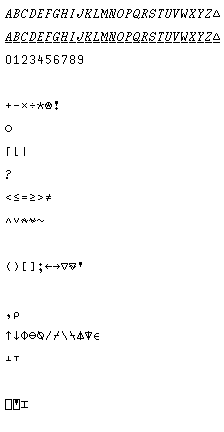[Next] [Up] [Previous] [Home] [Other]

# A Systematic Survey

The diagram below shows the APL characters used with APL\360:The first two lines show the alphabetic characters, that is, those that can be in the first position of a variable name. Note that in addition to the letters of the alphabet, the triangle symbol can be used in this way, and that these characters may be underscored.

The third line shows the digits, which may also occur in variable names in any position but the first.

Next, we see the basic mathematical operators. Plus, minus, times, and divide. The star denotes exponentiation, and the star with a circle gives the logarithm of its right argument to the base indicated by its left argument.

Unary plus is the identity function, and unary minus the additive inverse, as in conventional mathematics. Unary divide is the reciprocal. Unary star is e (2.71828...) to the power of its argument, and unary circle star, similarly, is the natural logarithm.

Unary multiply is the signum function; if its argument is negative, the result is -1; if positive, +1, if zero, zero.

Unary exclamation mark (and, incidentally, the exclamation mark in APL is the overstrike of the quotation mark and the period) is the factorial. (If the argument is fractional, the Gamma function of the argument minus one is calculated.) Dyadic exclamation point calculates the choose function; that is, a ! b is b!/(a! * (b-a)!).

On the next line we meet the circle function. This is used for the circular functions of trigonometry, and related functions.

1 circle a is sin(a), 2 circle a is cos(a), and 3 circle a is tan(a). And -1 circle a is arcsin(a), -2 circle a is arccos(a), and -3 circle a is arctan(a).

5 circle a is sinh(a), 6 circle a is cosh(a), 7 circle a is tanh(a), -5 circle a is inv sinh(a), -6 circle a is inv cosh(a), and -7 circle a is inv tanh(a).

0 circle a is sqrt(1-x^2); 4 circle a is sqrt(x^2+1); and -4 circle a is sqrt(x^2-1).

On the next line, we meet ceiling, floor, and absolute value. Ceiling rounds up, floor rounds down. The dyadic forms of ceiling and floor return the greater or lesser of their two arguments respectively. The dyadic vertical bar is used for the residue function; a | b is b mod a.

Again on a line by itself is the question mark, used for the roll and deal functions. ? a is a random integer from 1 to a; a ? b is a distinct random integers from 1 to b, and, thus, it can be used to shuffle cards in a game.

On the next line, we see the relational operators. These are dyadic only, with their conventional meanings; they return 0 for false and 1 for true.

After the relational operators come the logical operators, and, or, nand, nor, and not. Not is unary only, the others are dyadic only.

After another blank line, we see some characters with unique functions; parentheses, used for changing the order of evaluation; square brackets, which subscript arrays, and which can also be used to indicate the dimension on which some special operators act; the semicolon, used to separate array subscripts and mix literal and numerical values on an output line; and the symbols used for function definition and locked function definition, and finally the quote mark, used to form character constants.

After another blank line, we proceed to the operators that perform operations directly related to vectors and matrices. First, we see the comma, which performs ravel (unary) and catenate (dyadic), and rho, which either gives the shape of an array, or restructures one.

On the next line, we see take, drop, reverse/rotate, reverse/rotate along first dimension, compress, compress along first dimension, expand, expand along first dimension, grade up, grade down, and membership.

Then we see decode and encode, used for converting to and from radix representations.

Finally, after another space, we see the quad, used for conventional input of variable values, the quote quad, used for input of whatever is typed to a string (as with BASIC's LINE INPUT statement), and the I-beam, which provides a number of special items of system information.

[Next] [Up] [Previous] [Home] [Other]